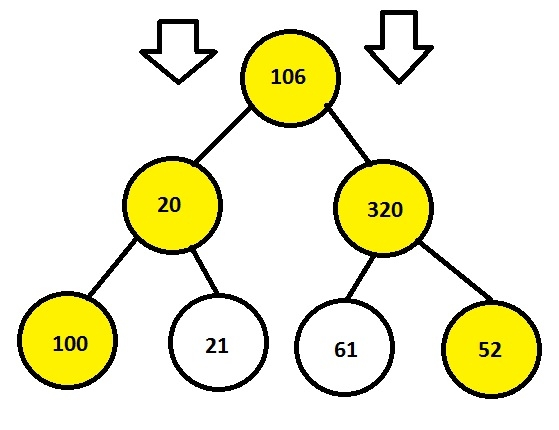# Print leftmost and rightmost nodes of a Binary Tree in C Program.

Given a binary tree with left and right children and the task is to print the exact right and left child of the given tree.

Leftmost nodes are the nodes which are associated on the left side from the parent node of the tree and rightmost nodes are which are associated on the right side from the parent node of the root.

## Example

Input: 106 20 320 100 21 61 52
Output: 106 20 320 100 52## Algorithm

Start
Step 1 -> create structure of a node
Declare int data
Declare struct node *left and *right
Step 2 -> create struct node* newNode(int val)
Create node* temp=new node
Set temp->data = val
Set temp->left = temp->right = NULL
return (temp)
step 3 -> Declare Function void print(node *root)
IF root == NULL
Return
Use STL queue<node*> que
Call que.push(root)
Use STL vector<int> ans
Loop While !que.empty()
Set int n = que.size()
Loop for int i =0 and i<n and i++
Set node *temp = que.front()
Set que.pop()
IF i=0
Set ans.push_back(temp->data)
End
Else IF i=n-1
Set ans.push_back(temp->data)
End
If temp->left
Set que.push(temp->left)
End
IF temp->right
Set que.push(temp->right)
End
End
Loop For auto i : ans
Print i
End
Step 4 -> In main()
Declare node *root = newNode(106) to create a node
Call print(root)
stop

## Example

#include <bits/stdc++.h>
using namespace std;
//structure of a node {
int data;
struct node* left, *right;
};
//structure to create a new node
struct node* newNode(int val){
node* temp = new node;
temp->data = val;
temp->left = temp->right = NULL;
return (temp);
}
//function to print corner elements of a tree
void print(node *root) {
if(root == NULL)
return;
queue<node*> que;
que.push(root);
vector<int> ans;
while(!que.empty()){
int n = que.size();
for(int i =0;i<n;i++){
node *temp = que.front();
que.pop();
if(i==0)
ans.push_back(temp->data);
else if(i==n-1)
ans.push_back(temp->data);
if(temp->left)
que.push(temp->left);
if(temp->right)
que.push(temp->right);
}
}
for(auto i : ans)
cout << i << " ";
}
int main (){
node *root = newNode(106);
root->left = newNode(20);
root->right = newNode(320);
root->left->left = newNode(100);
root->left->right = newNode(21);
root->right->left = newNode(61);
root->right->right = newNode(52);
print(root);
return 0;
}

## Output

if we run above program then it will generate following output

106 20 320 100 52